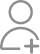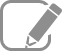Take Class 6 Tuition from the Best Tutors

•Affordable fees
•1-1 or Group class
•Flexible Timings
•Verified Tutors

Search in

# There are 20 girls and 15 boys in a class.(a) What is the ratio of number of girls to the number of boys?(b)What is the ratio of number of girls to the total number of students in the class?Follow 1AnswerNumber of girls = 20 Number of boys = 15 Total number of students = 20 + 15 = 35 (a) Ratio of number of girls to boys = (b) Ratio of number of girls to total students =

Number of girls = 20

Number of boys = 15

Total number of students = 20 + 15 = 35

(a) Ratio of number of girls to boys =(b) Ratio of number of girls to total students =Now ask question in any of the 1000+ Categories, and get Answers from Tutors and Trainers on UrbanPro.com

Find Class 6 Tuition near you

Looking for Class 6 Tuition ?

Learn from the Best Tutors on UrbanPro

Are you a Tutor or Training Institute?

Join UrbanPro Today to find students near you
X

### Looking for Class 6 Tuition Classes?

The best tutors for Class 6 Tuition Classes are on UrbanPro

• Select the best Tutor
• Book & Attend a Free Demo
• Pay and start Learning### Take Class 6 Tuition with the Best Tutors

The best Tutors for Class 6 Tuition Classes are on UrbanPro